出租广告位,需要合作请联系站长

+关注

2020-10(49)

2020-11(16)

## 摘要

1. 导入包创建图
``````import matplotlib.pyplot as plt
import pandas as pd
import numpy as np
fig = plt.figure()
ax.plot(np.random.randn(1000).cumsum())
```123456```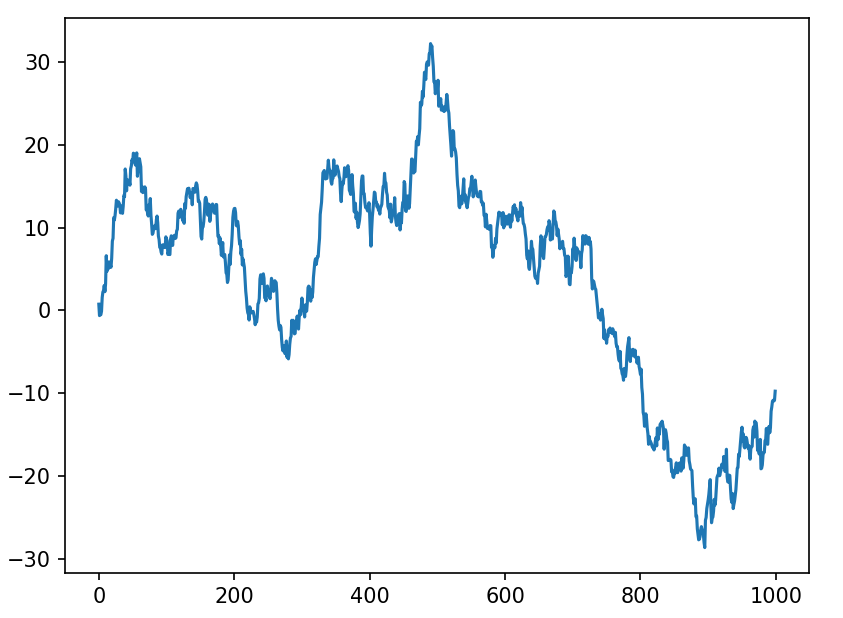2. 更改x轴刻度(set_xticks)

``````ax.set_xticks([0, 250, 500, 750, 1000])
```1```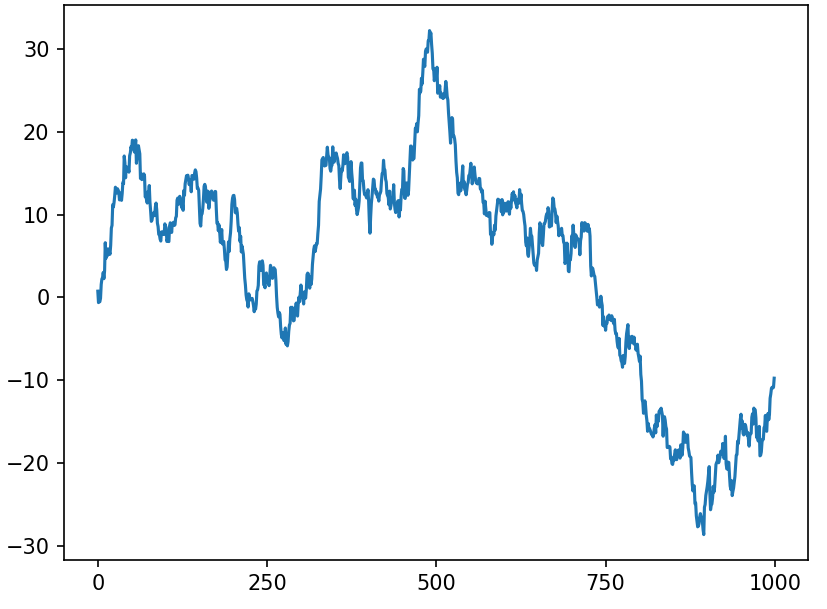3. 更改x轴刻度标签角度(rotation设置倾斜角度)

``````ax.set_xticklabels(['one', 'two', 'three', 'four','five'], rotation=30, fontsize='small')
```1```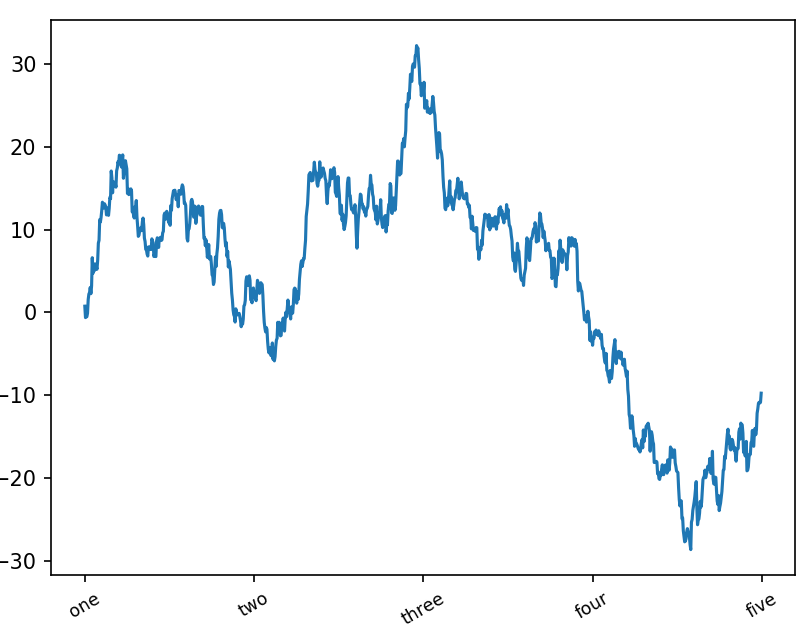4. 设置x轴标签(set_xlabel)

``````ax.set_xlabel('Stages')
```1```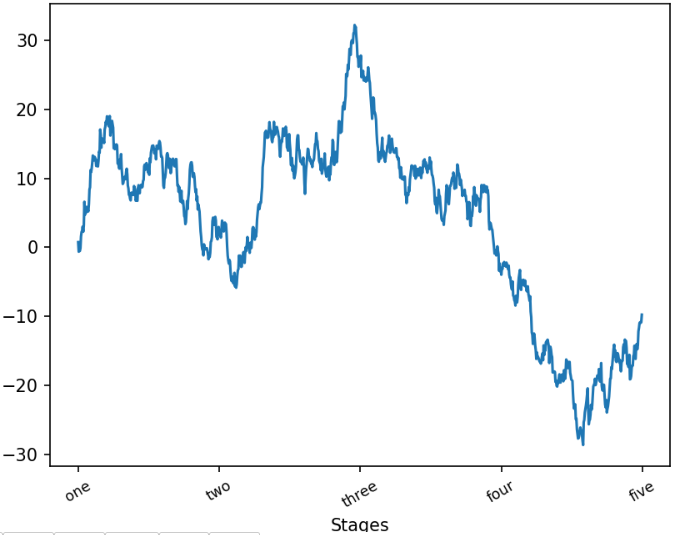5.设置标题

``````ax.set_title('My first matplotlib plot')
```1```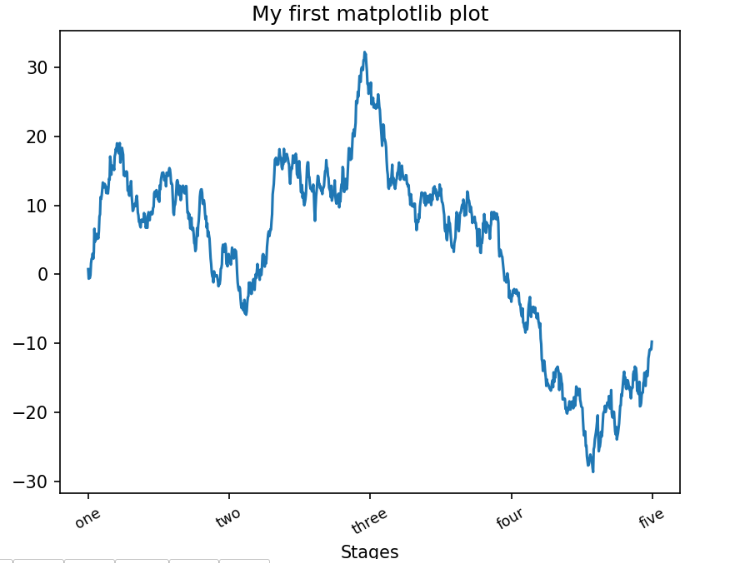0 0

pdf(new) 更多>